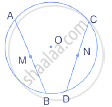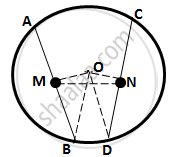Share

# M and N Are the Mid-points of Two Equal Chords Ab and Cd Respectively of a Circle with Centre O. Prove That: (I) ∠Bmn = ∠Dnm. (Ii) ∠Amn = ∠Cnm. - Mathematics

Course

#### Question

M and N are the mid-points of two equal chords AB and CD respectively of a circle with centre O. prove that:
(i) ∠BMN  = ∠DNM.
(ii) ∠AMN = ∠CNM.#### SolutionDrop OM⊥AB  and  ON⊥CD

∴ OM bisects AB and ON bisects CD
(Perpendicular drawn from the centre of a circle to a chord bisects it)

⇒ BM =1/2 AB = 1/2  CD =DN    .............(1)

Applying Pythagoras theorem,

OM2= OB2 -BM2

= OD2 - DN2          (by (1))
= ON2

∴ OM=ON

⇒  ∠OMN = ⇒ ∠ONM    ……………(2)

(Angles opp to equal sides are equal)

(i) ∠OMB=∠OND         (both 90°)

Subtracting (2) from above,

∠BMN= ∠DNM

(ii) ∠OMA = ONC         (both 90°)